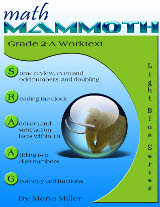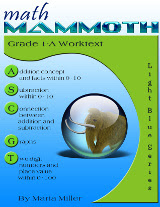## Posts

Showing posts from April, 2012

### See the first 4 million digits of PiThis online visualization represents the first 4,000,000 decimals of Pi within a single image. Each unique digit of Pi corresponds to a specific color, and is rendered as a 1x1 pixel dot. You can also search for the first occurrences of any specific decimal combination.

### Math Mammoth Grade 2 aligned to the Common Core StandardsMath Mammoth Grade 2 Complete Curriculum is now aligned to the Common Core Standards.

Here is a document listing the lessons and the corresponding standards.

I will record here the main changes in its content as compared to the earlier edition / version (through April 2012).

You can tell the new edition and the earlier ones apart by their cover image: the edition aligned to the Common Core Standards has these new cover images.

The topics of rounding and finding 1/4 of a number were taken off as they are not in Common Core Standards for grade 2.

The topics of regrouping in addition and regrouping in subtraction are now split so that 2-A contains regrouping in addition, and 2-B contains regrouping in subtraction.

There is now more explicit (more scaffolded) instruction about adding four single-digit numbers, and then adding four two-digit numbers in columns (in 2-A).

Regrouping in subtraction with three-digit numbers is also scaffolded more (the progression of the concept presentat…

### Math Mammoth Grade 1 aligned to the Common Core StandardsMath Mammoth Grade 1 Complete Curriculum is now aligned to the Common Core Standards.

Here is a document listing the lessons and the corresponding standards.

I will record here the main changes in its content as compared to the earlier edition / version (through April 2012).

You can tell the new edition and the earlier ones apart by their cover image: the edition aligned to the Common Core Standards has these new cover images.

What used to be chapter 4 (Place Value) switched places with Chapter 3 (Addition and Subtraction Facts within 0-18).

The topics of rounding, even & odd numbers, and parenthesis were taken off as they are  not in the Common Core standards for grade 1.

There are many new lessons about adding and subtracting within 20, and adding and subtracting two-digit numbers without regrouping, explaining basic  mental math principles.

The lesson "Exploring Measuring" is split into two lessons (Measuring Length and Exploring Measuring). Both lessons are expa…

### Geometry problems (challenges)

http://fivetriangles.blogspot.com/

It contains challenging geometry problems for middle and high school level. Unfortunately I didn't see solutions!

This blog for teachers contains an unordered series of problems for middle and junior high school students. The problems are not intended to be ``clever'', but rather require only a knowledge of already-learned basic mathematics principles, applied in a more sophisticated manner than for the problems found in a typical textbook.

### Fred and Frank running times puzzleHere's a puzzle for you to solve.

Fred and Frank are two fitness fanatics on a run from A to B. Fred runs half the way and walks the other half. Frank runs for half the time and walks for the other half. They both run and walk at the same speeds. Who finishes first?

Puzzle from Fawn Nguyen's puzzles and brainteasers, set 13

Here's one possible way to solve it. Choose some easy numbers for the distance they travel, for the speeds, and for the time in case of Frank.Then just calculate!

Let's say they run 10 miles per hour and walk 5 miles per hour. Let's also say the distance from A to B is 20 miles.

Fred runs half the way. So, Fred runs 10 miles = 1 h, and he walks 10 miles = 2 h. So he takes a total of 3 hours.

Frank runs half the time and walks half the time. Now, we don't know the time, so let it be t. We can write an equation about the total distance:

(distance running) + (distance walking) = 20 miles

(1/2t) * 10 + (1/2t) * 5 = 20

5t + 2.5t = 20

7.5 t = 20

t…

### Measuring videos

I've made a page filled with measuring-related videos, for about 4th grade level but you can use them with 3rd and 5th too.

Topics include measuring to the 1/8th of an inch, in millimeters & centimeters, conversions between inches, feet, and yards, metric units of weight, of length, and of volume, customary units of weight, temperature, and time.